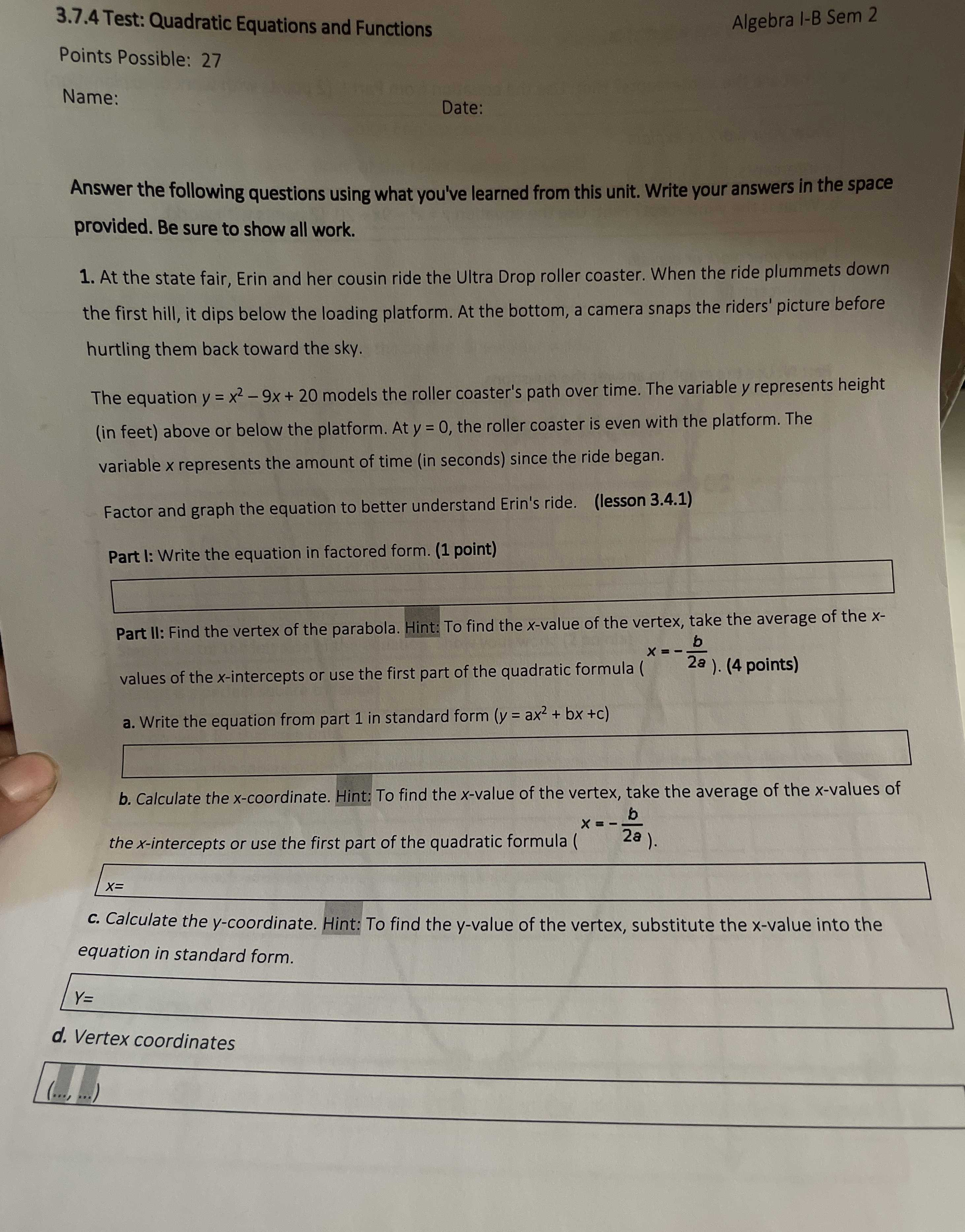### ¿Todavía tienes preguntas de matemáticas?

Pregunte a nuestros tutores expertos
Algebra
Pregunta1. At the state fair, Erin and her cousin ride the Ultra Drop roller coaster. When the ride plummets down the first hill, it dips below the loading platform. At the bottom, a camera snaps the riders' picture before hurtling them back toward the sky. The equation $$y = x ^ { 2 } - 9 x + 20$$ models the roller coaster's path over time. The variable y represents height (in feet) above or below the platform. At $$y = 0$$ , the roller coaster is even with the platform. The variable $$x$$ represents the amount of time (in seconds) since the ride began. Factor and graph the equation to better understand Erin's ride.

Part l: Write the equation in factored form.

Part II: Find the vertex of the parabola. Hint: To find the $$x$$ -value of the vertex, take the average of the $$x$$ - values of the $$x$$ -intercepts or use the first part of the quadratic formula ( $$x = - \frac { b } { 2 a } )$$

a. Write the equation from part $$1$$ in standard form $$( y = a x ^ { 2 } + b x + c )$$

b. Calculate the $$x$$ -coordinate. Hint: To find the $$x$$ -value of the vertex, take the average of the $$x$$ -values of the $$x$$ -intercepts or use the first part of the quadratic formula ( $$x = - \frac { b } { 2 a } )$$ .

c. Calculate the $$y$$ -coordinate. Hint: To find the $$y$$ -value of the vertex, substitute the $$x$$ -value into the equation in standard form. $$y =$$

d. Vertex coordinates

part I:

y=(x-4)(x-5)

Part II:

a) $$y= x^2- 9x+ 20$$

b) $$x= \frac{9}{2}$$

c) $$y= - \frac{1}{4}$$

d) $$(\frac{9}{2}, - \frac{1}{4})$$

Solución
View full explanation on CameraMath App.